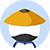At the beginning of January 2003, Elizabeth invested money in an account that collected interest, compounding more frequently than a year. Assume the annual percentage rate of interest remained constant. What is the total amount she has invested after seven years?

Statement #1: her initial investment was \$20,000

Statement #2: the account accrued 7% annual interest
• AStatement 1 ALONE is sufficient to answer the question, but statement 2 alone is NOT sufficient.
• BStatement 2 ALONE is sufficient to answer the question, but statement 1 alone is NOT sufficient.
• CBOTH statements 1 and 2 TOGETHER are sufficient to answer the question, but NEITHER statement ALONE is sufficient.
• DEach statement ALONE is sufficient to answer the question.
• EStatement 1 and 2 TOGETHER are NOT sufficient to answer the question.

• KMF解析
• 网友解析

### 题目讨论

• 优质讨论
• 最新讨论